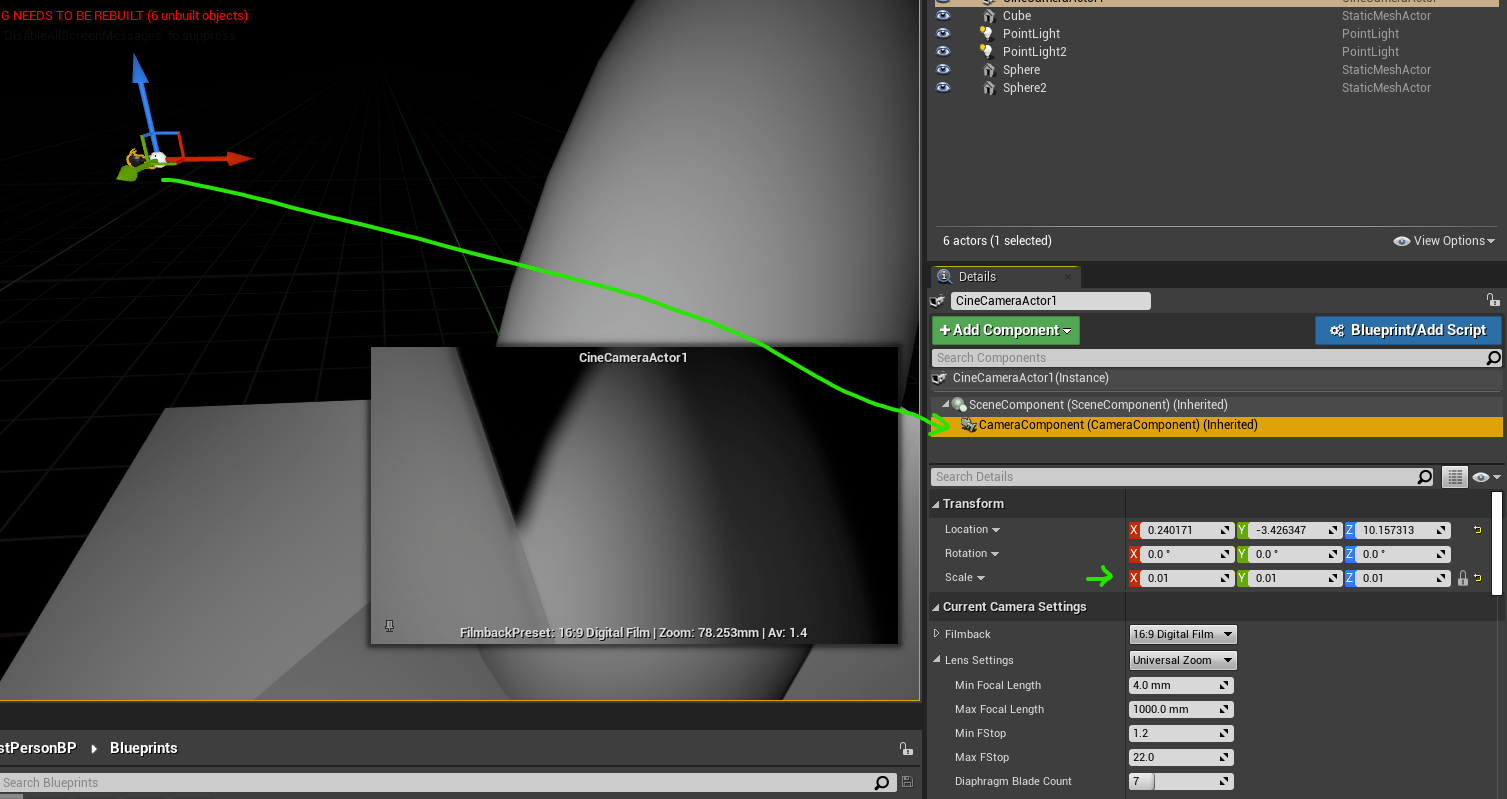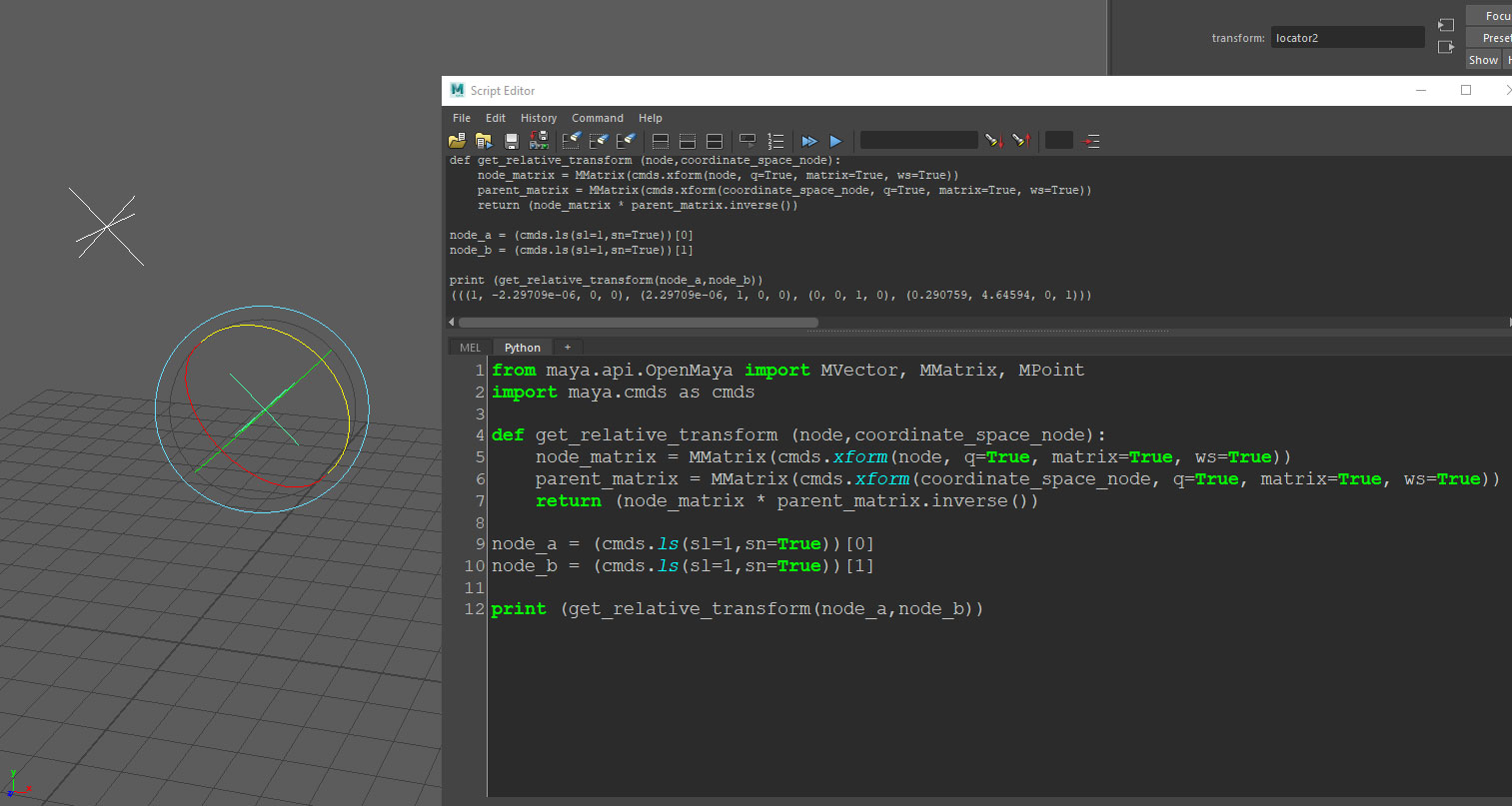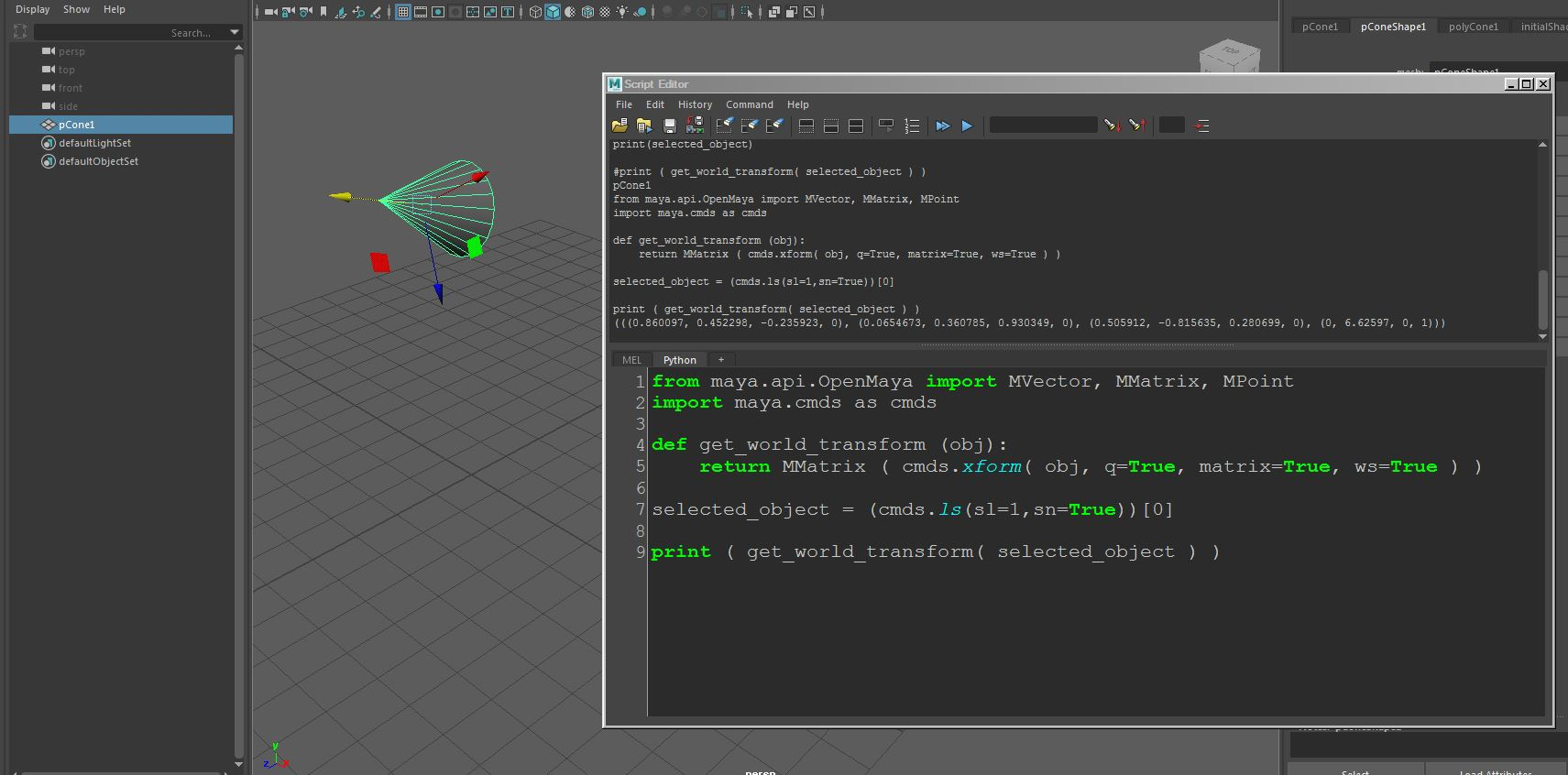## UE4 – Tiny tips for tiny scale projects

Software:
Unreal Engine 4.26

When having to develop a UE4 project that deals with a tiny world scale, like the whole level being less the 50cm size for example,
The following steps may help make the project more easily navigate-able and convenient to work on.

1. Scale down the camera Icon:
UE4 has by default a huge, bulky, opaque camera icon.
For a tiny scale project, this camera icon may cover the whole level and be very inconvenient to work with.
Select the relevant camera component and scale it down.
In my tests, this modified camera matrix isn’t breaking the camera optics in any way,
But if you want to have no such scale offsets in your project, you can also replace the camera icon with a suitable small one.2. In Editor Preferences > Viewport:
Decrease both:
Mouse Scroll Camera Speed and Mouse Sensitivity
To allow finer navigation at small scaleNote:
A global scale conversion factor can be used instead of taking these measures,
And in many cases this can be a more practical solution for managing a sub 50cm world,
For example, building everything 100X size so that 1 meter will be representing 1 centimeter in your project’s world.
But take into account, that if the project demands rendering realistic physical lighting and optics, extra conversions will have to be made to account for the scale conversion factor, so is such cases it may be better to setup a real-world scale project.

## Maya Python scripting – Getting an object’s transform matrix relative to another object’s coordinates

Software:
Maya 2018

How to get an object transformation matrix relative to another object’s coordinates:
* The following script requires selecting 2 objects, the function will return the transform matrix of the first object relative to the transform matrix of the second.

```from maya.api.OpenMaya import MVector, MMatrix, MPoint
import maya.cmds as cmds

def get_relative_transform (node,coordinate_space_node):
node_matrix = MMatrix(cmds.xform(node, q=True, matrix=True, ws=True))
parent_matrix = MMatrix(cmds.xform(coordinate_space_node, q=True, matrix=True, ws=True))
return (node_matrix * parent_matrix.inverse())

node_a = (cmds.ls(sl=1,sn=True))
node_b = (cmds.ls(sl=1,sn=True))

print (get_relative_transform(node_a,node_b))```## Maya Python scripting – Getting an Object’s world-space transform matrix

Software:
Maya 2018

How to get an object transformation matrix in world space coordinates:

```from maya.api.OpenMaya import MVector, MMatrix, MPoint
import maya.cmds as cmds

def get_world_transform (obj):
return MMatrix ( cmds.xform( obj, q=True, matrix=True, ws=True ) )

selected_object = (cmds.ls(sl=1,sn=True))

print ( get_world_transform( selected_object ) )```